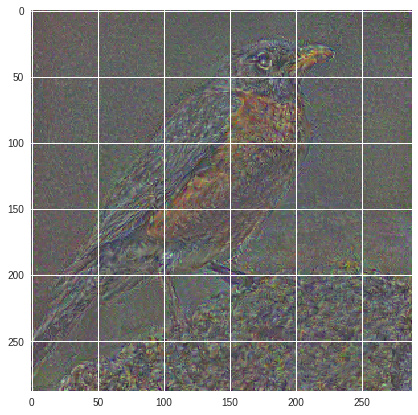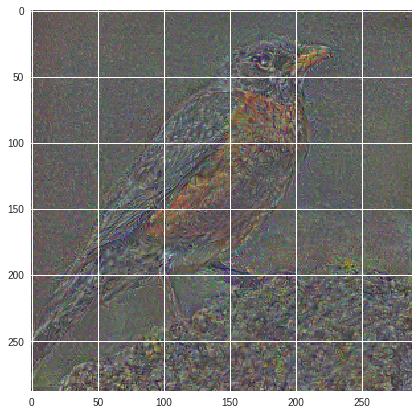# Idea: Loss normalization

I was watching this week lesson 12 and 13 and I’ve noticed how there is the need to move the loss in the same order of magnitude when there are multiple components in order to (smootly) train.

This morning I woke up with and idea to normalize the loss automatically (I havn’t tested it yet), but here is in short:

``````import numpy as np

loss1 = 0.000000001
loss2 = 1000.0

loss_exp1=np.log(loss1)
loss_exp2=np.log(loss2)

loss_norm1 = a1 * (np.e**(-loss_exp1))
loss_norm2 = a2 * (np.e**(-loss_exp2))

print('loss1:',loss1)
print('loss2:',loss2)

print('loss exp1:',loss_exp1)
print('loss exp2:',loss_exp2)

print('loss_norm1:',loss_norm1)
print('loss_norm2:',loss_norm2)
``````

Output:

``````loss1: 1e-09
loss2: 1000.0
loss exp1: -20.72326583694641
loss exp2: 6.907755278982137
loss_norm1: 0.9999999999999982
loss_norm2: 1.0000000000000007
``````

My 2 centsAll right I’ve run some test on the style transfer notebook ( which is a bit special as it uses LFBGS, so I’ll need to test it on other optimizers too ) but, the results are encouraging, with some drawbacks.

I’ve tested it by making a clone of the random image and optimize the content loss only
It turned out to be in general a just little bit slower than a fixed factor (*1000) but it saves you time by not requiring to fiddle around to find with values.

Loss *1000 fixed factor:

``````Iteration: 100, loss: 0.00021629194088745862
Iteration: 200, loss: 9.297583892475814e-05
Iteration: 300, loss: 6.070972085581161e-05
Iteration: 400, loss: 4.6014258259674534e-05
Iteration: 500, loss: 3.7611818697769195e-05
Iteration: 600, loss: 3.220088183297776e-05
Iteration: 700, loss: 2.845807102858089e-05
Iteration: 800, loss: 2.5683024432510138e-05
Iteration: 900, loss: 2.348573980270885e-05
Iteration: 1000, loss: 2.1716399714932777e-05
``````

Normalized Loss:

``````Iteration: 100, loss: 0.00024975594715215266
Iteration: 200, loss: 0.00010198856034548953
Iteration: 300, loss: 6.494418630609289e-05
Iteration: 400, loss: 4.915898898616433e-05
Iteration: 500, loss: 4.0430750232189894e-05
Iteration: 600, loss: 3.437769919401035e-05
Iteration: 700, loss: 3.0084884201642126e-05
Iteration: 800, loss: 2.7044028684031218e-05
Iteration: 900, loss: 2.4746024791966192e-05
Iteration: 1000, loss: 2.28744866035413e-05
``````

Loss *1000 fixed factor:Normalized Log Loss:( to be continued )